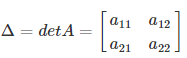# Square Matrix

Matrix is one of the most commonly used elements in linear algebra. Matrix is the rectangular arrangement of numbers/elements/objects. The horizontal arrangement is called the row and the vertical arrangement is the column of a matrix. The order of a matrix is determined by the number of rows by columns. Suppose a matrix has 2 rows and 3 rows of elements, then its order is 2×3. In the same way, when a matrix has an equal number of rows and columns, then the matrix is called the square matrix. In this article, you will learn the mathematical definition of a square matrix, properties of square matrix and examples in detail.

Learn: Matrices

## Square Matrix Definition

A square matrix is a matrix that has an equal number of rows and columns. In mathematics, m × m matrix is called the square matrix of order m. If we multiply or add any two square matrices, the order of the resulting matrix remains the same.

Square Matrix Example

$$\begin{array}{l}X=\left[\begin{array}{lll}2 & -7 & 7 \\ 2 & 5 & 6 \\ 5 & 4 & 3\end{array}\right]\end{array}$$

In the above example, we can see, the number of rows and columns are three respectively. Since the order of the matrix is 3 × 3, hence X is a square matrix. We can also find the determinant of a 3×3 square matrix here.

Again, if we take another example, such as;

$$\begin{array}{l}Y=\left[\begin{array}{lll}9 & 5 & 7 \\ 3 & 8 & 9\end{array}\right]\end{array}$$

Here, the number of rows is two and the number of columns is 3. Since rows and columns of matrix Y are not equal, hence it is not a square matrix.

### Square Matrix of Order 2

The square matrix of order 2 is the matrix with 2 rows and 2 columns. The general form of a 2×2 matrix or the square matrix of order 2 is given by:

$$\begin{array}{l}A =\begin{bmatrix} a_{11} &a_{12} \\ a_{21}&a_{22} \end{bmatrix}\end{array}$$

### Square Matrix of Order 3

A square matrix of order 3 contains 3 rows and 3 columns, that means its order is 3×3. The general form of square matrix with order 3 is given as:

$$\begin{array}{l}A =\begin{bmatrix} a_{11} &a_{12} & a_{13}\\ a_{21}&a_{22} & a_{23}\\ a_{31}&a_{32}&a_{33} \end{bmatrix}\end{array}$$

As we have already mentioned in the introduction part, that two square matrices can be added in a very simple way. Let us consider there is 3 by 3 matrix A and B whose values are given here:

$$\begin{array}{l}\begin{array}{l}\left[\begin{array}{lll}a_{11} & a_{12} & a_{13} \\ a_{21} & a_{22} & a_{23} \\ a_{21} & a_{22} & a_{23}\end{array}\right]+\left[\begin{array}{lll}b_{11} & b_{12} & b_{13} \\ b_{21} & b_{22} & b_{23} \\ b_{21} & b_{22} & b_{13}\end{array}\right]=\left[\begin{array}{llll}a_{11}+b_{11} & a_{12}+b_{12} & a_{13}+b_{13} \\ a_{21}+b_{21} & a_{22}+b_{22} & a_{23}+b_{23} \\ a_{31}+b_{21} & a_{32}+b_{12} & a_{33}+b_{23}\end{array}\right] \\ For Example:\left[\begin{array}{lll}1 & 2 & 3 \\ 4 & 5 & 6 \\ 7 & 8 & 9\end{array}\right]+\left[\begin{array}{ccc}10 & 11 & 12 \\ 13 & 14 & 15 \\ 16 & 17 & 18\end{array}\right]=\left[\begin{array}{ccc}1+10 & 2+11 & 3+12 \\ 4+13 & 5+14 & 6+15 \\ 7+16 & 8+17 & 9+18\end{array}\right] \\ =\left[\begin{array}{ccc}11 & 13 & 15 \\ 17 & 19 & 21 \\ 23 & 25 & 27\end{array}\right]\end{array}\end{array}$$

As we can see from the above example, the addition of square matrices is very important to perform. Each value in one matrix of a row is added to the other value of the same row and column of another matrix.

## Multiplication of Square Matrix

Just like the addition method, the two square matrices are multiplied in the simple way. Let us consider two 2 by 2 square matrices to be multiplied together. Hence, the resultant matrix will be:

$$\begin{array}{l}\begin{array}{l}\left[\begin{array}{lll}a_{11} & a_{12} \\ a_{21} & a_{22} \end{array}\right]+\left[\begin{array}{lll}b_{11} & b_{12} \\ b_{21} & b_{22} \end{array}\right]=\left[\begin{array}{llll}a_{11}b_{11}+ a_{12}b_{12} & a_{11}b_{12}+ a_{12}b_{22} \\ a_{21}b_{11}+a_{22}b_{21} & a_{21}b_{12}+a_{22}b_{22} \end{array}\right] \end{array}\end{array}$$

Note: The number of rows and columns should always be the same.

### Square Matrix Determinant

The determinant of a matrix is the scalar value or a number estimated using a square matrix. The square matrix could be any number of rows and columns such as 2×2, 3×3, 4×4, or in the form of n × n, where the number of columns and rows are equal.

Check: Determinant of a Matrix

If S is the set of square matrices, R is the set of numbers (real or complex) and f : S → R is defined by f (A) = k, where A ∈ S and k ∈ R, then f (A) is called the determinant of A. The determinant of a square matrix A is denoted by “det A” or | A |.

For a 2 by 2 matrix, the determinant is given by:det A = a11×a22-a12×a21

Hence, we can find the determinant using this formula.

### Square Matrix Properties

Some of the important properties of square matrices are listed below:

• The number of rows and columns is equal.
• The sum of all the diagonal elements of a square matrix is called the trace of a matrix.
• If all the diagonal elements of a square matrix are equal to 1, then it is called an identity matrix.
• For a square matrix, we can perform different operations such as inverse.
• The determinant value can be calculated only for the square matrix.
• The order of transpose of a square matrix is the same as the original matrix.